summary of site-wide JavaScript functionalityUnited States-English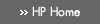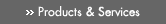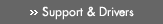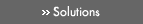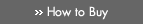» Contact HPSearch:More optionsManualTechnical documentation - EnglishAll of HP USHP-UX Reference > Part IV Section 3: Library Functions# L»

## Technical documentation» Feedback## » Indexl64a(3C) - convert between long integer and base-64 ASCII string — see a64l(3C)
l64a_r(3C) - convert between long integer and base-64 ASCII string — see a64l(3C)
labs(3C) - return integer absolute value — see abs(3C)
lcong48(3C) - generate uniformly distributed pseudo-random numbers — see drand48(3C)
lcong48_r(3C) - generate uniformly distributed pseudo-random numbers — see drand48(3C)
ldcvt(3C) - convert long-double floating-point number to string
ldecvt(3C) - convert long-double floating-point number to string — see ldcvt(3C)
ldecvt_r(3C) - convert long-double floating-point number to string — see ldcvt(3C)
ldexp(3M) - scale exponent of a floating-point number
ldexpf(3M) - scale exponent of a floating-point number — see ldexp(3M)
ldexpl(3M) - scale exponent of a floating-point number — see ldexp(3M)
ldexpq(3M) - scale exponent of a floating-point number — see ldexp(3M)
ldexpw(3M) - scale exponent of a floating-point number — see ldexp(3M)
ldfcvt(3C) - convert long-double floating-point number to string — see ldcvt(3C)
ldfcvt_r(3C) - convert long-double floating-point number to string — see ldcvt(3C)
ldgcvt(3C) - convert long-double floating-point number to string — see ldcvt(3C)
ldgcvt_r(3C) - convert long-double floating-point number to string — see ldcvt(3C)
ldiv(3C) - integer division and remainder — see div(3C)
leaveok(3X) - terminal output control functions — see clearok(3X)
lfind(3C) - linear search and update — see lsearch(3C)
lgamma(3M) - log gamma functions
lgamma_r(3M) - log gamma functions — see lgamma(3M)
lgammaf(3M) - log gamma functions — see lgamma(3M)
lgammaf_r(3M) - log gamma functions — see lgamma(3M)
lgammal(3M) - log gamma functions — see lgamma(3M)
lgammal_r(3M) - log gamma functions — see lgamma(3M)
lgammaq(3M) - log gamma functions — see lgamma(3M)
lgammaq_r(3M) - log gamma functions — see lgamma(3M)
lgammaw(3M) - log gamma functions — see lgamma(3M)
lgammaw_r(3M) - log gamma functions — see lgamma(3M)
libcom_err(3) - Kerberos client libraries — see libkrb5(3)
libcom_err.sl(3) - Kerberos client libraries — see libkrb5(3)
libcom_err.so(3) - Kerberos client libraries — see libkrb5(3)
libIO(3X) - interface for interacting with kernel I/O subsystem
libio(3X) - interface for interacting with kernel I/O subsystem — see libIO(3X)
libk5crypto(3) - Kerberos client libraries — see libkrb5(3)
libk5crypto.sl(3) - Kerberos client libraries — see libkrb5(3)
libk5crypto.so(3) - Kerberos client libraries — see libkrb5(3)
libkrb5(3) - Kerberos client libraries
libkrb5.sl(3) - Kerberos client libraries — see libkrb5(3)
libkrb5.so(3) - Kerberos client libraries — see libkrb5(3)
libslp(3N) - SLP (Service Location Protocol) library routines
LINES(3X) - number of lines on terminal screen
llabs(3C) - return integer absolute value — see abs(3C)
lldiv(3C) - integer division and remainder — see div(3C)
llrint(3M) - round to nearest long long functions
llrintf(3M) - round to nearest long long functions — see llrint(3M)
llrintl(3M) - round to nearest long long functions — see llrint(3M)
llrintq(3M) - round to nearest long long functions — see llrint(3M)
llrintw(3M) - round to nearest long long functions — see llrint(3M)
llround(3M) - round to long long functions
llroundf(3M) - round to long long functions — see llround(3M)
llroundl(3M) - round to long long functions — see llround(3M)
llroundq(3M) - round to long long functions — see llround(3M)
llroundw(3M) - round to long long functions — see llround(3M)
localeconv(3C) - query the numeric formatting conventions of the current locale
localtime(3C) - convert date and time to string — see ctime(3C)
localtime_r(3C) - convert date and time to string — see ctime(3C)
log(3M) - natural logarithm functions
log10(3M) - common logarithm functions
log10f(3M) - common logarithm functions — see log10(3M)
log10l(3M) - common logarithm functions — see log10(3M)
log10q(3M) - common logarithm functions — see log10(3M)
log10w(3M) - common logarithm functions — see log10(3M)
log1p(3M) - natural logarithm of one-plus-argument functions
log1pf(3M) - natural logarithm of one-plus-argument functions — see log1p(3M)
log1pl(3M) - natural logarithm of one-plus-argument functions — see log1p(3M)
log1pq(3M) - natural logarithm of one-plus-argument functions — see log1p(3M)
log1pw(3M) - natural logarithm of one-plus-argument functions — see log1p(3M)
log2(3M) - logarithm base two functions
log2f(3M) - logarithm base two functions — see log2(3M)
log2l(3M) - logarithm base two functions — see log2(3M)
log2q(3M) - logarithm base two functions — see log2(3M)
log2w(3M) - logarithm base two functions — see log2(3M)
logbf(3M) - radix-independent exponent functions — see logb(3M)
logbl(3M) - radix-independent exponent functions — see logb(3M)
logbq(3M) - radix-independent exponent functions — see logb(3M)
logbw(3M) - radix-independent exponent functions — see logb(3M)
logf(3M) - natural logarithm functions — see log(3M)
logl(3M) - natural logarithm functions — see log(3M)
logname(3C) - return login name of user
logq(3M) - natural logarithm functions — see log(3M)
logw(3M) - natural logarithm functions — see log(3M)
longjmp(3C) - non-local goto — see setjmp(3C)
longname(3X) - get verbose description of current terminal
lrand48(3C) - generate uniformly distributed pseudo-random numbers — see drand48(3C)
lrand48_r(3C) - generate uniformly distributed pseudo-random numbers — see drand48(3C)
lrint(3M) - round to nearest long int functions
lrintf(3M) - round to nearest long int functions — see lrint(3M)
lrintl(3M) - round to nearest long int functions — see lrint(3M)
lrintq(3M) - round to nearest long int functions — see lrint(3M)
lrintw(3M) - round to nearest long int functions — see lrint(3M)
lround(3M) - round to long int functions
lroundf(3M) - round to long int functions — see lround(3M)
lroundl(3M) - round to long int functions — see lround(3M)
lroundq(3M) - round to long int functions — see lround(3M)
lroundw(3M) - round to long int functions — see lround(3M)
lsearch(3C) - linear search and update
ltoa(3C) - convert long integers to strings — see ltostr(3C)
ltoa_r(3C) - convert long integers to strings — see ltostr(3C)
ltostr(3C) - convert long integers to strings
ltostr_r(3C) - convert long integers to strings — see ltostr(3C)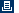Printable versionPrivacy statement Using this site means you accept its terms Feedback to webmaster© 1983-2007 Hewlett-Packard Development Company, L.P.